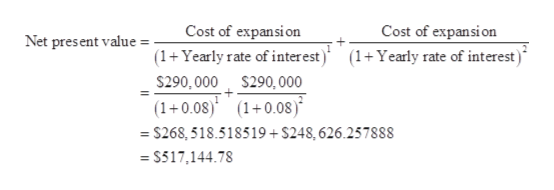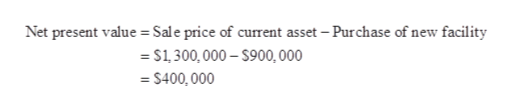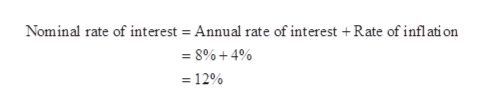# Optimal corporation wants to expand their manufacturing facilities. They have two choices, first to expand the current site at a cost of \$290,000 per year for two years to complete the expansion, or to sell their current site for \$1.3 million and purchase a new larger facility at a cost of \$900,000 in the industrial zone.If the annual interest rate is 8%, evaluate the cash flows for the two options described above and decide which is the most financially beneficial to the corporation?If Optimal corporation wants to factor inflation in their calculations, what is the equivalent nominal interest rate if expected inflation rate is 4% in the coming years? Critically discuss the significance of including the factor of inflation in corporate finance calculations

Question
9 views

Optimal corporation wants to expand their manufacturing facilities. They have two choices, first to expand the current site at a cost of \$290,000 per year for two years to complete the expansion, or to sell their current site for \$1.3 million and purchase a new larger facility at a cost of \$900,000 in the industrial zone.

1. If the annual interest rate is 8%, evaluate the cash flows for the two options described above and decide which is the most financially beneficial to the corporation?
2. If Optimal corporation wants to factor inflation in their calculations, what is the equivalent nominal interest rate if expected inflation rate is 4% in the coming years? Critically discuss the significance of including the factor of inflation in corporate finance calculations
check_circle

Step 1

1. The net present value of option 1:

Computation of net present value:

Hence, the net present value in option 1 is \$517,144.78.help_outlineImage TranscriptioncloseCost of expansi on Cost of expansion Net present value . (1+Yearly rate of inter est) (1+Yearly rate of interest) \$290, 000 \$290, O00 (1+0.08) (10.08) =\$268,518.518519+ \$248, 626.257888 \$517,144.78 fullscreen
Step 2

The net present value of option 2:

Computation of net present value:

Hence, the net present value in option 2 is \$400,000.help_outlineImage TranscriptioncloseNet present value Sale price of current asset - Purchase of new facility S1,300,000-\$900, 000 S400, 000 fullscreen
Step 3

2. Computation of nominal rate of interest:

Hence, the nominal rate of interest is 12%.

...help_outlineImage TranscriptioncloseNominal rate of interest Annual rate of interest +Rate of inflati on =8%+4% =12% fullscreen

### Want to see the full answer?

See Solution

#### Want to see this answer and more?

Solutions are written by subject experts who are available 24/7. Questions are typically answered within 1 hour.*

See Solution
*Response times may vary by subject and question.
Tagged in